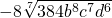# 9.2 Reducing Higher Power Roots

While square roots are the most common type of radical, there are higher roots of numbers as well: cube roots, fourth roots, fifth roots, and so on. The following is a definition of radicals: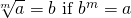The small letter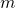inside the radical is called the index. It dictates which root you are taking. For square roots, the index is 2, which, since it is the most common root, is not usually written.

Example 9.2.1

Here are several higher powers of positive numbers and their roots: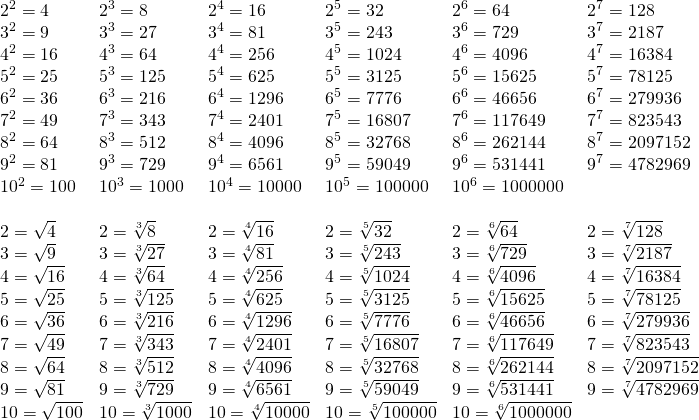Note there is a notable distinction between solutions of even roots and of odd roots. For even-powered roots, the solution is always +/− or ±. The reason for this can shown in the following examples.

Example 9.2.2

Find the solutions to √4.

There are two ways to multiple identical numbers to equal 4: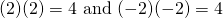This means that the √4 is either +2 or −2, which is often written as ±2.

The ± solution occurs for all even roots and can be seen in: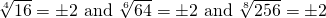All roots that have an even index will always have ± solutions.

Odd-powered roots do not share this feature and will only maintain the sign of the number that you are taking the root of.

Example 9.2.3

Find the solutions to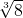and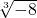.

The solution ofis 2 andis −2.

The reason for this is (2)3 = 8 and (−2)3 = −8.

All negative-indexed roots will keep the sign of the number being rooted.

Higher roots can be simplified in much the same way one simplifies square roots: through using the product property of radicals.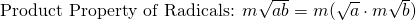Examples 9.2.4

Use the product property of radicals to simplify the following.

1.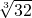32 can be broken down into 25. Since you are taking the cube root of this number, you can only take out numbers that have a cube root. This means that 32 is broken into 8 × 4, with the number 8 being the only number that you can take the cube root of.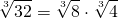This simplifies to: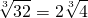2.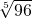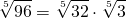96 can be broken down into 25 × 3. Since you are taking the fifth root of this number, you can only take out numbers that have a fifth root. This means that 96 is broken into 32 × 3, with the number 32 being the only number that you can take the fifth root of.This simplifies to: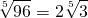This strategy is used to take the higher roots of variables. In this case, only take out whole number multiples of the root index. This is shown in the following examples.

Example 9.2.5

Reduce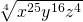.

For this root, you will break the exponent into multiples of the index 4.

This means that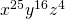will be broken up into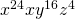.

The fourth roots of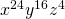are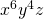and the solitary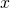remains under the fourth root radical. This looks like: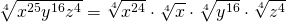Which simplifies to: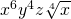Example 9.2.6

Reduce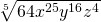.

For this root, you will break the exponent into multiples of the index 5.

This means thatwill be broken up into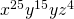and 64 broken up into 32 × 2.

The fifth roots of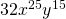are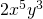and the remainder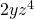remains under the fifth root radical.

This looks like: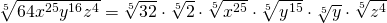Which simplifies to: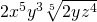# Questions

1.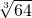2.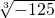3.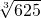4.5.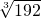6.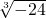7.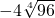8.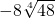9.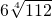10.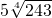11.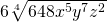12.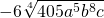13.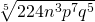14.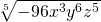15.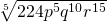16.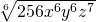17.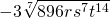18.